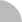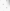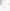# 臺灣博碩士論文加值系統

(34.204.172.188) 您好！臺灣時間：2023/09/26 05:12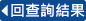:::

### 詳目顯示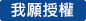: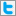Twitter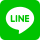•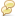被引用:0
•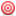點閱:170
•評分: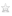•下載:0
•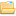書目收藏:1
 在這篇論文中，我們考慮流形上的非線性發展方程。在第一章，我們介紹一些有關黎曼度量的保角形變及子流形的移動等問題，這些是近幾年來我們所關心的課題。 在第二章，首先我們用博柯納（Bochner）公式、質量遞減的估計和橢圓型的莫澤（Moser）疊代，證明了３-維緊流形在具有任意的黎曼度量的卡拉比流（Calabi flow）的長時間的存在性。其次，我們證明了對３-維緊流形的卡拉比流存在其解的子序列的漸進收斂。作為它的應用，我們驗證了在３-維緊流形上其常曲率的二次泛函的臨界度量的存在性，此可以視為３-維緊流形的堯麻比（Yamabe）問題的推廣。 在第三章，我們證明了在二維完備歐氏空間上修正里奇流（modified Ricci flow）的漸展的常曲率的哈那克（Harnack）估計，在一些適當的曲率假設之下。 在第四章，我們證明了在四維完備歐氏空間上，當對起始拉格朗日環曲面（Lagrangian torus）T0² 有一些適當的假設之下，則拉格朗日環曲面（Lagrangian tori）Tt² 的形狀在平均曲率流(mean curvature flow)作用之下，很快地趨近於乘積環曲面（product torus）的形狀。特別地，在有限的時間內其拉格朗日環曲面Tt² 未崩潰成一點前不會產生奇異點。
 In this thesis, we consider nonlinear evolution equations on manifolds. In chapter 1, we introduce some problems of the deformation of Riemannian metrics and the motion of submanifolds which my research concerned in the past few years. In chapter 2, based on Bochner formula, mass decay estimates and elliptic Moser iteration, we first show the global existence of the 3-dimensional Calabi flow on any closed 3-manifold with an arbitrary background metric g0. Second, we show the asymptotic convergence of a subsequence of solutions of the Calabi flow on a closed 3-manifold. With its application, we prove the existence of extremal metrics for quadratic functional of scalar curvature on a closed 3-manifold which is served as an extension of the Yamabe problem on closed manifolds. In chapter 3, we prove the Harnack estimate for the evoloved curvature R of the modified Ricci flow on complete R² under some curvature assumptions. In chapter 4, we show that, under suitable assumptions for a initial Lagrangian torus T0², the shape of Lagrangian tori Tt² in R4 under the mean curvature flow approaches the shape of a product torus very rapidly. In particular, no singularities will develop before the Lagrangian tori Tt² collapse to a point in a finite time.
 1. Introduction ………………………………………………….....2 2. The Calabi flow on closed 3-manifolds ……………………. 5 2.1 Introduction ………………………………………………. 5 2.2 The mass decay estimate ………………………………… 8 2.3 A priori estimate and long time existence …………17 2.4 Find a uniformly lower bound ………………………… 23 2.5 Asymptotic convergence to an extremal metric …….27 3. The modified Ricci flow on complete R² …………………. 33 3.1 Introduction ……………………………………………….33 3.2 Basic properties of The modified Ricci flow ………35 3.3 The Harnack quantity …………………………………….39 3.4 The trace Harnack estimate …………………………….41 3.5 The Matrix Harnack stimate …………………………….46 4. Mean curvature flow of Lagrangian tori in R4 ……………56 Reference ……………………………………………………….......60
 [Au] T. Aubin, Nonlinear Analysis on Manifolds, Monge-Ampère Equations, Die Grundlehren der Math. Wissenschaften, Vol.252, Springer-Verlag, New York, 1982.[Be] A. Besse, Einstein Manifolds, Springer-Verlag, New York, 1986.[Ca] E. Calabi, Extremal Kähler Metrics, Seminars on Differential Geometry (S.T. Yau, ed.), Princeton Univ. Press and Univ. of Tokyo Press, Princeton, New York, 1982, pp.259-290.[Ch] B. Chow, The Yamabe flow on locally conformally flat manifolds with positive Ricci curvature, Commun. Pure and Appl. Math., XLV (1992), 1003-1014.[Cho] ---------, The Ricci flow on 2-sphere, J. Diff. Geom. 33(1991), 325-334.[CHW] S.-C. Chang, S.-K. Hong and C.-T. Wu, The Harnack estimate for the modified Ricci flow on complete R², to appear in Rocky Mountain J. of Math.[CW1] S.-C. Chang, C.-T. Wu, Mean curvature flow of Lagrangian tori in R4, preprint.[CW2] ---------, Extremal metrics for quadratic functional of scalar curvature on complete noncompact 3-manifolds, in preparation.[CW3] ---------, Extremal metrics for quadratic functional of scalar curvature on closed 3-manifolds, preprint.[CW4] ---------, On the existence of extremal metrics for L²-norm of scalar curvature on closed 3-manifolds, J. of Mathematics Kyoto University, Vol. 39, No 3 (1999), 435-454.[Ch1] S.-C. Chang, Critical Riemannian 4-Manifolds, Math. Z. 214 (1993), 601-625.[Ch2] ---------, A Harnack Estimate for The Calabi Flow on closed 4-Manifolds, preprint.[Ch3] ---------, The Calabi Flow on Riemann Surfaces, preprint.[Ch4] ---------, The Calabi Flow on Einstein Manifolds,Lectures on Analysis and Geometry( S.T. Yau ed.) International Press, Hong Kong, 1997, pp. 29-39.[Ch5] ---------, Existence and Convergence of Solutions of Non-linear Evolution Equations on 4-manifolds, Lectures on Analysis and Geometry( S.T. Yau ed.) International Press, Hong Kong, 1997, pp. 41--50.[Ch6] ---------, On the Existence of Nontrivial Extremal Metrics on Complete Noncompact Surfaces, Math. Ann. (to appear).[Ch7] ---------, Global Existence and Convergence of Solutions of Calabi Flow on Surfaces, J. of Math. Kyoto University Vol. 40, No. 2 (2000), 363-377.[Ch8] ---------, Global Existence and Convergence of Solutions of the Calabi Flow on Einstein \$4\$-Manifolds, Nagoya Math. J. Vol. 163 (2001), 193-214.[Ch9] ---------, Compactness Theorems and The Calabi Flow on Kaehler Surfaces with Stable Tangent Bundle, Math. Ann.318 (2000), 315-340.[Cha] I. Chavel, Eigenvalues in Riemannian Geometry, Academic Press, New York, 1984.[Chr] P.T. Chruściel, Semi-Global Existence and Convergence of solutions of the Robinson-Trautman (2-Dimensional Calabi) Equation, Commun. Math. Phys. 137 (1991), 289-313.[CY1] S.-Y. A. Chang, P. Yang, Compactness of Isospectral Conformal Metrics on S³, Comment. Math. Helvetici 64 (1989), 363-374.[CY2] ---------, Isospectral Conformal Metrics on 3-manifolds, J. Ameri. Math. Soc. 3 (1990), 117-145.[Cr] C.B. Croke, Some Isoperimetric Inequalities and Eigenvalue Estimates, Ann. Sci. Ec. Norm. Super. 13 (1980), 419-435.[De] D. DeTurck, Deforming Metrics in the Direction of Their Ricci Tensors, J. Differential Geom. 18 (1983), 157-162.[GH] M.Gage and R.S. Hamilton, The Shrinking of Convex Plane Curves by Heat Equation, J. Diff. Geom. 23 (1986), 69-96.[Gr] M. Grayson, Shortening embedded curves, Ann. of Math, 129 (1989), 71-111.[Gu] M. J. Gursky, Compactness of Conformal Metrics with Integral Bounds on Curvature, Duke Math. J. Vol. 72, No. 2 (1993), 339-367.[H1] R.S. Hamilton, Three-manifolds with positive Ricci curvature, J. Diff. Geom. 17 (1982), 255-306.[H2] ---------, The Ricci flow on surface, Mathematics and General Relativity, J. Isenberg, ed., Contemp. Math. Vol. 71, AMS, 1988, 237-262.[H3] ---------, The Harnack estimate for the Ricci flow, J. Diff. Geom. 37 (1993), 225-243.[Hu] G. Huisken, Flow by Mean Curvature of Convex Surfaces into Spheres, J. Diff. Geom. 20 (1984), 237-26[HS] G. Huisken and C. Sinestrari, Mean Curvature Flow Singularities for Mean Convex Surfaces, Calc. Var. 8(1999), 1-14.[Ka] J.L. Kazdan, Deformation to Positive Scalar Curvature on Complete Manifolds, Math. Ann. 261 (1982), 227-234.[KW] J.L. Kazdan, F.W. Warner, Existence and Conformal Deformation of Metrics with Prescribed Gaussian and Scalar Curvatures, Ann. of Math. 101 (2) (1975), 317-331.[Lu] A. Lunardi, Asymptotic Exponential Stability in Quasilinear Parabolic Equations, Nonlin. Anal. 9 (1985), 563-586.[LT] P. Li, L. -F. Tam, The Heat Equation and Harmonic Maps of Complete Manifolds, Invent. Math. 105 (1991), 1-46.[Sh1] W.-X. Shi, Deforming the metric on complete noncompact Riemannian manifolds, J. Diff. Geom. 30 (1989), 223-301.[Sh2] ---------,Ricci deformation of the metric on complete noncompact Riemannian manifolds, J. Diff. Geom. 30 (1989), 303-394.[Si] L. Simon, Asymptotics for a Class of Nonlinear Evolution Equations, with Applications to Geometric Problems, Ann. of Math., 118 (1983), 525-571.[Sm] K. Smoczyk, A canonical Way to Deform a Lagrangian Submanifold, preprint 1996.[SY] R. Schoen & S.-T. Yau, Lectures on Differential Geometry, International Press, 1994.[Wa1] M.-T. Wang, Mean curvature flow of surfaces in Einstein 4-manifolds, to appear in J.D.G.[Wa2] ---------, Deforming area preserving diffeomorphism of surfaces by mean curvature flow, preprint.[W1] C.-T. Wu, The modified Ricci flow on complete R², in preparation.[Wu] L.-F. Wu, The Ricci flow on complete R², Commun. in Analysis and Geometry, Vol. 1, No. 3 (1993), 439-472.[Zh] J. Zhiren, A Counterexample to the Yamabe Problem for Complete Noncompact Manifolds, Lecture Notes in Mathematics, 1306, Springer-Verlag, Berlin, 1988.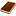國圖紙本論文推文當script無法執行時可按︰推文 網路書籤當script無法執行時可按︰網路書籤 推薦當script無法執行時可按︰推薦 評分當script無法執行時可按︰評分 引用網址當script無法執行時可按︰引用網址 轉寄當script無法執行時可按︰轉寄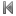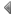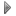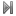top
 無相關論文

 無相關期刊

 1 流形上的幾何拋物型方程 2 流氣上的幾何拋物型方程 3 半線性橢圓方程正解的存在性與多樣性 4 中文建議語在團體諮商之運用 5 台灣華語介音歸屬的實驗研究 6 分組問題與斯特靈數的探討 7 優生救國---潘光旦思想析論 8 臺灣的阿片想像：從舊慣的阿片君子，到更生院的矯正樣本 9 虛雲禪師（1840？∼1959）與近代中國佛教 10 男色興盛與明清的社會文化 11 從台銀與美援資金分配探討公民營事業之發展（1945-1965） 12 上半平面模型中的一些工具 13 P-Laplacian邊界爆炸值問題分歧曲線漸進行為之研究 14 關於獨立隨機數列的完全收斂之研究 15 關於兩個物種對於三個相配資源競爭的數學模型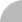簡易查詢 | 進階查詢 | 熱門排行 | 我的研究室